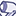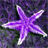# QlikView App Dev

Discussion Board for collaboration related to QlikView App Development.

Announcements
Join this live chat April 6, 10AM EST - QlikView to Qlik Sense REGISTER
cancel
Showing results for
Search instead for
Did you mean:Creator

## Tolerance variables

Hi,

I have a straight table where I calculate the variance of the the cost value from the mean.  To do this I use the following expression:

avg(EXTENDED_AMT*CONVERSION_RATE) / avg(total <COST_CD> EXTENDED_AMT*CONVERSION_RATE)-1

I am trying to restrict the output in the table if the value returned in the above is > 20%.

I tried to do this using an input box and the following expression, but it didn't work:

//if( (avg(EXTENDED_AMT*CONVERSION_RATE) / avg(total <COST_CD> EXTENDED_AMT*CONVERSION_RATE)) > vToleranceLevel, (avg(EXTENDED_AMT*CONVERSION_RATE)) / avg(total <COST_CD> EXTENDED_AMT*CONVERSION_RATE),0)

avg(EXTENDED_AMT*CONVERSEION_RATE) / avg(total <COST_CD> EXTENDED_AMT*CONVERSION_RATE)-1

vToleranceLevel is my input variable.

Any help will be much appreciated.

Regards,

Daniel

2 RepliesPartner

Hi.

I'd start by doing those multiplications on your script.

EXTENDED_AMT*CONVERSION_RATE as NewField1,

To restrict the number of records or values to be shown, they are some ways.

If is a straight table, do it in the dimension limits tab.

You can use calculated dimensions in the dimension tab.

You can notice them with visual clues or other color changes.

Send an example. Easy, with little data, to see what you have in your table.

Salutes.Creator
Author

Hi Christian,

Thank you for your reply.

Ideally I would like to be have the choice of the following:

1) An input box to set the level of variance from the mean of to be shown in the table

2) An expression/dimension that restricts the level of variance from the mean to be shown in the table

I have a test qvw file, but I am not sure how to attach it on this forum?

Any help with the above would be great.

Thanks,

Daniel